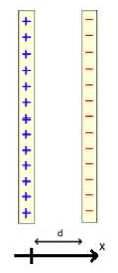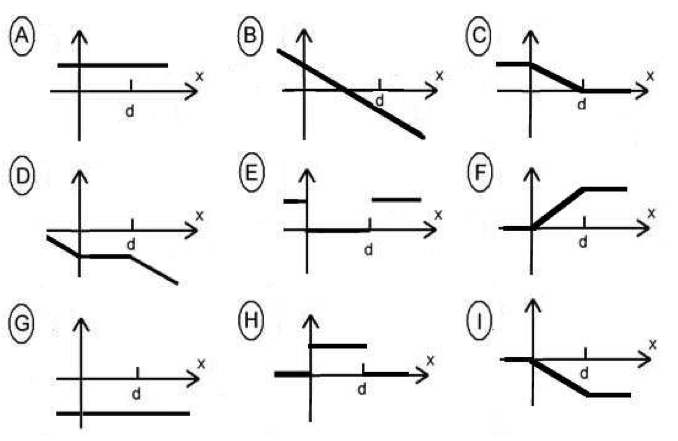Teaching Physics with the Physics Suite Edward F. Redish Problems Sorted by Type | Problems Sorted by Subject | Problems Sorted by Chapter in UP

Sheets of charge

 Two large parallel sheets of charge are separated by a distance d , small compared to the size of the sheets. The distance d is small enough that the sheets can be treated as if they were infinite in extent. The charge per unit area on the sheets are +σ and -σ respectively. For this problem, we are considering the electric field and potential along the coordinate line x shown. The 0 of the coordinate is at the left sheet. A. Select which graphs below could be appropriate as a graph of each of the following quantities as a function of x . If more than one answer works, give them all. If none works, write N. (a) The electric field (b) The electrostatic potential Explain your reasoning.B. If the distance between the sheets is halved, does either the potential or the electric field between the sheets change? Explain.

Not finding what you wanted? Check the Site Map for more information.